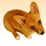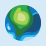# Azimuth between two map points?

2673
2
12-29-2015 07:22 AMNew Contributor III

Any way to return azimuth between two map points?

2 RepliesFrequent Contributor

You may want to check out the GeometryEngine.GeodesicDistance Method

GeometryEngine.GeodesicDistance Method

 Calculates the geodesic distance between 2 given points and calculates the azimuth at both points for the geodesic curves that connects the points.New Contributor
 Chris Smith wrote:You may want to check out the GeometryEngine.GeodesicDistance MethodGeometryEngine.GeodesicDistance MethodCalculates the geodesic distance between 2 given points and calculates the azimuth at both points for the geodesic curves that connects the points.

That is the documentation for GeometryEngine.GeodesicDistance Method but from the syntax, there doesn't seem to be any way of retrieving the azimuth.

 public static double GeodesicDistance(MapPoint point1,MapPoint point2,LinearUnit unit)

Am I missing something?